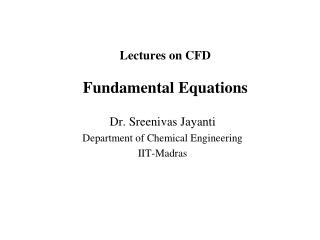Download PresentationLectures on CFD Fundamental Equations

# Lectures on CFD Fundamental Equations - PowerPoint PPT PresentationDownload Presentation## Lectures on CFD Fundamental Equations

- - - - - - - - - - - - - - - - - - - - - - - - - - - E N D - - - - - - - - - - - - - - - - - - - - - - - - - - -
##### Presentation Transcript

1. Lectures on CFDFundamental Equations Dr. Sreenivas Jayanti Department of Chemical Engineering IIT-Madras

2. Equations Solved in CFD • Conservation of mass † • Conservation of linear momentum† • Conservation of energy • Equation of state • Initial and boundary conditions † Mass and momentum conservation equations together are usually called Navier-Stokes equations

3. Governing Equations for Incompressible, Constant Property Flow • Continuity equation : • Momentum conservation equation: • Energy conservation equation:

4. Outline of the Finite Volume Method • The CFD approach • Discretization of the governing equations • Converts each partial differential equation into a set of coupled algebraic equations

5. THE CFD APPROACH • Assembling the governing equations • Identifying flow domain and boundary conditions • Geometrical discretization of flow domain • Discretization of the governing equations • Incorporation of boundary conditions • Solution of resulting algebraic equations • Post-solution analysis and reformulation, if needed

6. J+1 j+1 J P - cell j J-1 I-1 i i-1 I i+1 I+1 I-2 V - cell U - cell THE STAGGERED GRID

7. CASE STUDY (Hand-calculation) Fully Developed Flow through a Triangular Duct

8. A Simple Example • Fully developed laminar flow in a triangular duct of irregular cross-section • Flow governing equation is known: boundary condition: w =0 on walls • Analytical solution not available for an arbitrary triangle • Velocity field can be readily obtained using CFD approach

9. A Simple Example: The CFD Solution • Governing equation put in conservation form: • Domain divided into triangles and rectangles • GE integrated over a control volume and is converted into a surface integral using Gauss’ Divergence Theorem: • Apply to each cell: • Each cell gives an algebraic equation linking the cell value with those of the neighbouring cells

10. The CFD Solution: Spatial Discretization Divide the domain into cells and locate nodes at which the velocity has to be determined The example below 20 nodes out of which 8 are boundary nodes with zero velocity; velocity at the other 12 needs to be calculated

11. The CFD Solution: Discretization of Equation

12. The CFD Solution: All the Equations • Application to all the cells gives a set of algebraic equations • In this case, 12 simultaneous linear algebraic equations

13. The CFD Solution: Set of Algebraic Equations • Put in a matrix form Aw =b and solve using standard methods to get wi

14. The CFD Solution • Solution of Aw =b, say, using Gauss-Seidel iterative method, gives the required velocity field.

15. The CFD Solution: Variants • To get more accuracy, divide into more number of cells and apply the same template of CFD solution

16. A Simple Example: The CFD Solution • At some point, the CFD solution becomes practically insensitive to further refining of the grid and we have a grid-independent CFD solution • Using the hydraulic diameter concept, one would have obtained a Reynolds number of 500 => an error of 23.8% if one goes by hydraulic diameter!